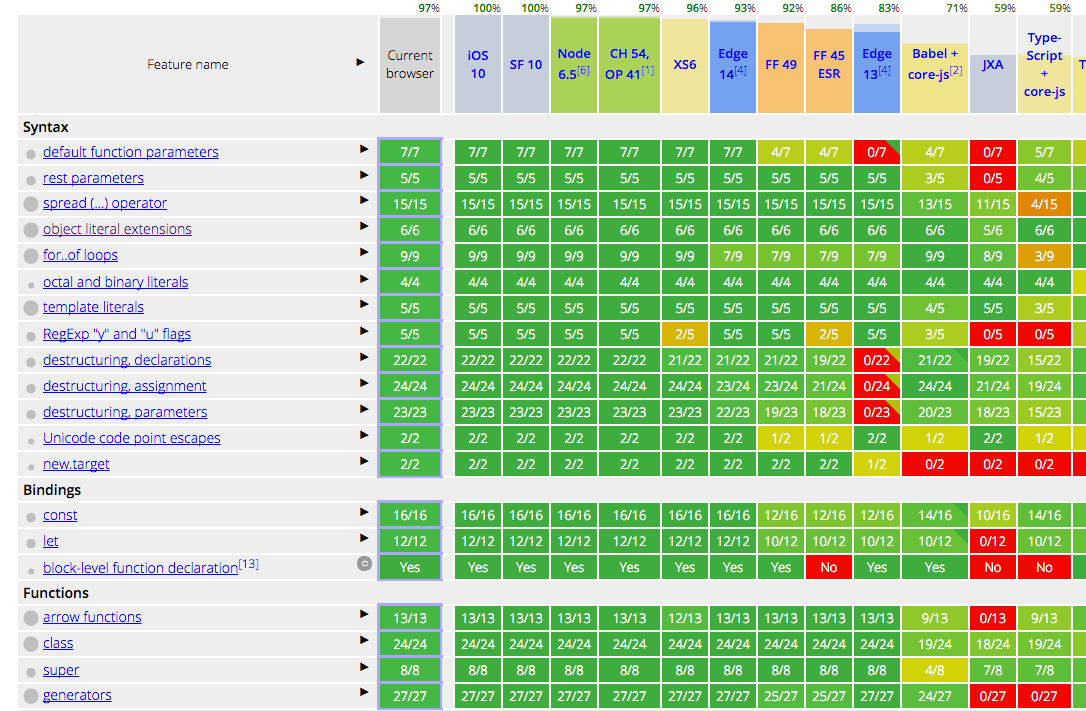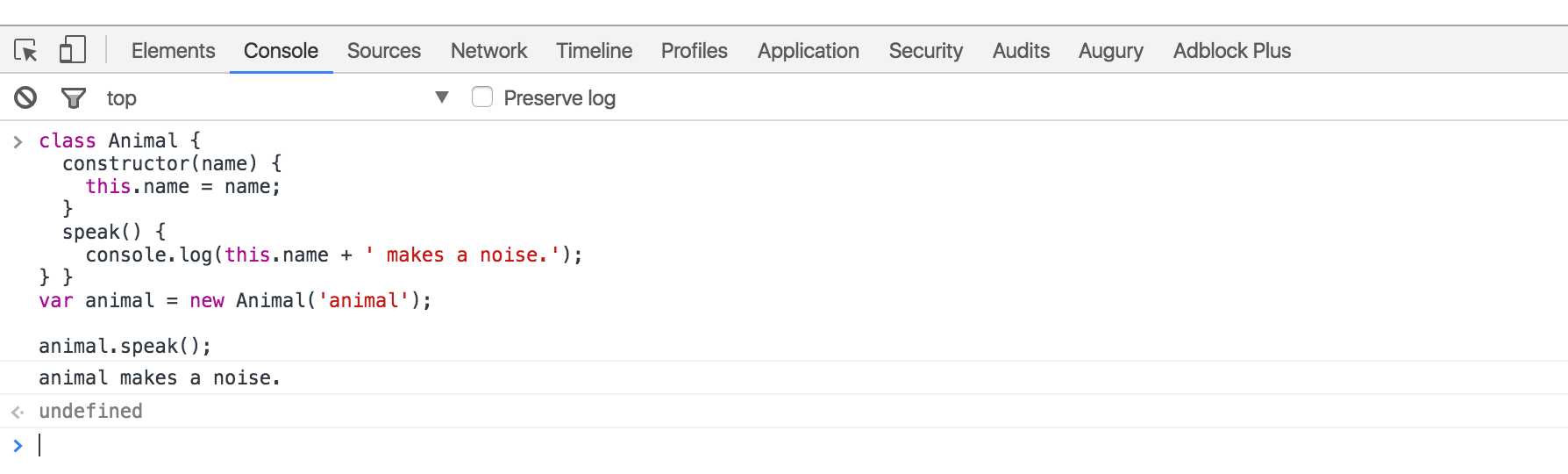karen

## JavaScript ES6功能概述（也称为ECMAScript 6和ES2015 +）

JavaScript在去年新增了12个特性可以使用。Chrome，MS Edge，Firefox，Safari，Node和许多其他环境已经内置支持JavaScript ES6的大部分功能。 因此，在本教程中你学习的所有知识都可以马上开始应用。`var`有什么问题吗？

`var` 的问题是变量泄漏到其他代码块中，例如`for`循环或`if`语句。

``````ES5
var x = 'outer';
function test(inner) {
if (inner) {
var x = 'inner';// scope whole function
return x;
}
return x;// gets redefined because line 4 declaration is hoisted
}
test(false);// undefined 😱
test(true);// inner

``````

`test(false)` 按理说会返回 `outer`， 但是不是的， 它返回了`undefined`

• `var` 是函数作用域。即使在声明之前，它在整个函数中也可用。

• 声明被提升，您可在它被声明之前使用一个变量。

• 初始化不会提升， 如果您使用`var`，则始终在顶部声明变量。

• 应用了提升规则后，我们更好得了解发生了什么：

``````
``````

ES5 var x = 'outer'; function test(inner) {
var x;// HOISTED DECLARATION
if (inner) {
x = 'inner';// INITIALIZATION NOT HOISTED
return x; }
return x; }

``````
``````

ECMAScript 2015 找到了解决办法：

``````ES6
let x = 'outer';
function test(inner) {
if (inner) {
let x = 'inner';
return x;
}
return x;// gets result from line 1 as expected
}
test(false);// outer
test(true);// inner

``````

`var`更改为`let`使代码按预期工作。 如果未调用`if`块，变量`x`将不会在代码块外被提升。

Let 提升 和 “暂时性死区”

• 在ES6中, `let`会把变量提升到块的顶部(而不是像ES5这样的函数顶部)。

• 不过，在变量声明之前在代码块中引用会导致`ReferenceError`错误。

• `let`是块级作用域。不能在它被声明之前引用。

• “暂时性死区” 是从代码块开始到变量声明为止的区域。

IIFE（立即执行函数表达式）

``````ES5
{
var private = 1;
}
console.log(private);// 1

``````

``````ES5
(function(){
var private2 = 1;})();
console.log(private2);// Uncaught ReferenceError

``````

ES6写法简化了很多， 我们可以只用代码块和`let`，不再需要使用IIFE：

``````ES6
{
let private3 = 1;
}
console.log(private3);// Uncaught ReferenceError

``````

Const常量• 对所有引用使用`const`；避免使用 `var`

• 如果需要重新指定引用，使用`let` 代替 `const`

``````ES5
var last = 'Mejia';
console.log('Your name is ' + first + ' ' + last + '.');

``````

``````const first = 'Adrian';
const last = 'Mejia';

``````

``````var template = '<li *ngFor="let todo of todos" [ngClass]="{completed: todo.isDone}" >\n' +
'  <div class="view">\n' +
'    <input class="toggle" type="checkbox" [checked]="todo.isDone">\n' +
'    <label></label>\n' +
'    <button class="destroy"></button>\n' +
'  </div>\n' +
'  <input class="edit" value="">\n' +
'</li>';
console.log(template);

``````

``````const template = `<li *ngFor="let todo of todos" [ngClass]="{completed: todo.isDone}" >
<div class="view">
<input class="toggle" type="checkbox" [checked]="todo.isDone">
<label></label>
<button class="destroy"></button>
</div>
<input class="edit" value="">
</li>`;
console.log(template);

``````

ES6的解构非常实用和简洁。如下例所示：

``````ES5
var array = [1, 2, 3, 4];
var first = array;
var third = array;
console.log(first, third);// 1 3

``````

``````ES6
const array = [1, 2, 3, 4];
const [first, ,third] = array;
console.log(first, third);// 1 3

``````

``````ES5
var a = 1;
var b = 2;
var tmp = a;
a = b;
b = tmp;
console.log(a, b);// 2 1

``````

``````ES6
let a = 1;
let b = 2;
[a, b] = [b, a];
console.log(a, b);// 2 1

``````

``````ES5
function margin() {
var left=1, right=2, top=3, bottom=4;
return {
left: left, right: right, top: top, bottom: bottom };
}
var data = margin();
var left = data.left;
var bottom = data.bottom;
console.log(left, bottom);// 1 4

``````

``````return [left, right, top, bottom];

``````

``````var left = data;
var bottom = data;

``````

``````ES6
function margin() {
const left=1, right=2, top=3, bottom=4;
return { left, right, top, bottom };
}
const { left, bottom } = margin();
console.log(left, bottom);// 1 4

``````

``````ES5
var user = {
};
function getFullName(user) {
var firstName = user.firstName;
var lastName = user.lastName;
return firstName + ' ' + lastName;
}

``````

``````ES6
const user = {
};
function getFullName({
firstName, lastName
}) {  return \${firstName} \${lastName};}

``````

``````ES5
function settings() {
return { display: { color: 'red' }, keyboard: { layout: 'querty'} };}
var tmp = settings();
var displayColor = tmp.display.color;var keyboardLayout = tmp.keyboard.layout;
console.log(displayColor, keyboardLayout);// red querty

``````

``````ES6
function settings() {
return { display: { color: 'red' }, keyboard: { layout: 'querty'} };
}
const {
display: { color: displayColor }, keyboard: { layout: keyboardLayout }
} = settings();
console.log(displayColor, keyboardLayout);// red querty

``````

• 使用数组解构来获取元素或交换变量。 它可以避免创建临时引用。

• 不要对多个返回值使用数组解构，而应使用对象解构。

``````ES5
var Animal = (function () {
function MyConstructor(name) {    this.name = name;
}
MyConstructor.prototype.speak = function speak() {    console.log(this.name + ' makes a noise.');
};
return MyConstructor;})();
var animal = new Animal('animal');
animal.speak();// animal makes a noise.

``````

``````ES6
class Animal {
constructor(name) {    this.name = name;}
speak() {    console.log(this.name + ' makes a noise.');
}
}
const animal = new Animal('animal');
animal.speak();// animal makes a noise.

``````

• 始终使用`class`语法，并避免直接操作`原型`。 为什么？ 因为它使代码更简洁，更易于理解。

• 避免使用空的构造函数。 如果未指定，则类具有默认的构造函数。

``````ES5
var Lion = (function () {
function MyConstructor(name){
Animal.call(this, name);
}
// prototypal inheritance
MyConstructor.prototype = Object.create(Animal.prototype);
MyConstructor.prototype.constructor = Animal;
MyConstructor.prototype.speak = function speak() {
Animal.prototype.speak.call(this);
console.log(this.name + ' roars 🦁');
};
return MyConstructor;})();
var lion = new Lion('Simba');lion.speak();// Simba makes a noise.
// Simba roars.

``````

• 第3行中，我们用参数显式调用`Animal`构造函数。

• 在7-8行，我们将Lion原型指派给Animal的原型。

• 第11行，我们从父类`Animal`中调用 `speak`方法。

``````class Lion extends Animal {
speak() {    super.speak();
console.log(this.name + ' roars 🦁');
}}
const lion = new Lion('Simba');
lion.speak();// Simba makes a noise.
// Simba roars.

``````

• `extends`内置方法实现继承。

``````ES5
function printAfterTimeout(string, timeout, done){
setTimeout(function(){
done(string);
}, timeout);}
printAfterTimeout('Hello ', 2e3, function(result){
console.log(result);// nested callback
console.log(result);
});
});

``````

``````ES6
function printAfterTimeout(string, timeout){
return new Promise((resolve, reject) => {
setTimeout(function(){      resolve(string);
}, timeout);
});}
printAfterTimeout('Hello ', 2e3).then((result) => {
console.log(result);
}).then((result) => {
console.log(result);
});

``````

ES6并未移除函数表达式，但添加了一个新的函数，即箭头函数。

``````ES5
var _this = this;// need to hold a reference
\$('.btn').click(function(event){
_this.sendData();// reference outer this
});
\$('.input').on('change',function(event){
this.sendData();// reference outer this
}.bind(this));// bind to outer this

``````

``````ES6
// this will reference the outer one
\$('.btn').click((event) =>  this.sendData());
// implicit returns
const ids = [291, 288, 984];
const messages = ids.map(value => `ID is \${value}`);

``````

`for``forEach` 再到 `for...of`

``````ES5
// for
var array = ['a', 'b', 'c', 'd'];
for (var i = 0;i < array.length;i++) {
var element = array[i];
console.log(element);}// forEach
array.forEach(function (element) {  console.log(element);});

``````

ES6 的for…of同样适用于循环迭代。

``````ES6
// for ...of
const array = ['a', 'b', 'c', 'd'];
for (const element of array) {
console.log(element);
}

``````

``````ES5
function point(x, y, isFlag){
x = x || 0;
y = y || -1;
isFlag = isFlag || true;
console.log(x,y, isFlag);
}
point(0, 0) // 0 -1 true 😱
point(0, 0, false) // 0 -1 true 😱😱
point(1) // 1 -1 true
point() // 0 -1 true

``````

• 第8行，我们传入 `0, 0`返回`0, -1`

• 第9行，我们传入 `false` 但是返回了`true`

``````ES6
function point(x = 0, y = -1, isFlag = true){
console.log(x,y, isFlag);
}
point(0, 0) // 0 0 true
point(0, 0, false) // 0 0 false
point(1) // 1 -1 true
point() // 0 -1 true

``````

``````ES5
function point(x, y, isFlag){
x = x || 0;
y = typeof(y) === 'undefined' ? -1 : y;
isFlag = typeof(isFlag) === 'undefined' ? true : isFlag;
console.log(x,y, isFlag);
}
point(0, 0) // 0 0 true
point(0, 0, false) // 0 0 false
point(1) // 1 -1 true
point() // 0 -1 true

``````

``````ES5
function printf(format) {
var params = [].slice.call(arguments, 1);
console.log('params: ', params);
console.log('format: ', format);
}

``````

``````ES6
function printf(format, ...params) {
console.log('params: ', params);
console.log('format: ', format);
}

``````

`apply()` 到扩展运算符。 我们同样用 `...` 来解决：``````ES5
Math.max.apply(Math, [2,100,1,6,43]) // 100

``````

``````ES6
Math.max(...[2,100,1,6,43]) // 100

``````

``````ES5
var array1 = [2,100,1,6,43];
var array2 = ['a', 'b', 'c', 'd'];
var array3 = [false, true, null, undefined];
console.log(array1.concat(array2, array3));

``````

``````ES6
const array1 = [2,100,1,6,43];
const array2 = ['a', 'b', 'c', 'd'];
const array3 = [false, true, null, undefined];
console.log([...array1, ...array2, ...array3]);

``````